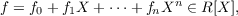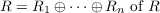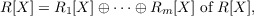#### Vol. 78, No. 1, 1978

 Recent Issues Vol. 325: 1 Vol. 324: 1  2 Vol. 323: 1  2 Vol. 322: 1  2 Vol. 321: 1  2 Vol. 320: 1  2 Vol. 319: 1  2 Vol. 318: 1  2Online Archive Volume: Issue:The Journal Subscriptions Editorial Board Officers Contacts Submission Guidelines Submission Form Policies for Authors ISSN: 1945-5844 (e-only) ISSN: 0030-8730 (print) Special Issues Author Index To Appear Other MSP Journals
On the divisors of monic polynomials over a commutative ring

### Robert William Gilmer, Jr. and William James Heinzer

Vol. 78 (1978), No. 1, 121–131
##### Abstract

For a commutative ring R with identity, denote by RXthe quotient ring of R[X] with respect to the regular multiplicative system S of monic polynomials over R. The present paper determines the group of units of the ring RX. This is equivalent to the problem of determining the saturation S of the multiplicative system S; by definition, S consists of all divisors of monic polynomials over R. For a nonzero polynomialit is shown that each of the following conditions (A) and (B) is equivalent to the condition that f divides a monic polynomial over R (in (B), the ring R is reduced).

1. The coefficients of f generate the unit ideal of R and, for each j between 0 and n and for each prime ideal P of R, the relations fj+1,,fn P, fjP, imply that fj is a unit modulo P.
2. There exists a direct sum decompositionsuch that if f = g1 + + gm is the decomposition of f with respect to the induced decompositionthen the leading coefficient of gi is a unit of Ri for each i.

One corollary to the preceding characterizations is that S is the set of polynomials over R with unit leading coefficient if and only if the ring R is reduced and indecomposable.

Primary: 13F20
##### Milestones